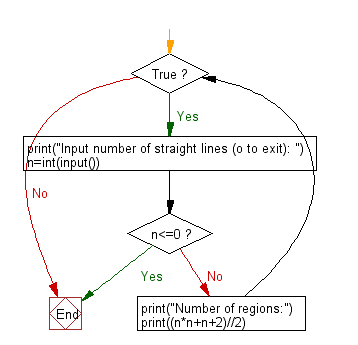﻿ Python: Create maximum number of regions obtained by drawing n given straight lines - w3resource# Python: Create maximum number of regions obtained by drawing n given straight lines

## Python Basic - 1: Exercise-54 with Solution

if you draw a straight line on a plane, the plane is divided into two regions. For example, if you pull two straight lines in parallel, you get three areas, and if you draw vertically one to the other you get 4 areas.
Write a Python program to create maximum number of regions obtained by drawing n given straight lines.

Input:
(1 ≤ n ≤ 10,000)
Input number of straight lines (o to exit):
5
Number of regions:
16

Sample Solution:

Python Code:

``````while True:
print("Input number of straight lines (o to exit): ")
n=int(input())
if n<=0:
break
print("Number of regions:")
print((n*n+n+2)//2)
``````

Sample Output:

```Input number of straight lines (o to exit):
5
Number of regions:
16
```

Flowchart:Python Code Editor:

Have another way to solve this solution? Contribute your code (and comments) through Disqus.

What is the difficulty level of this exercise?

Test your Programming skills with w3resource's quiz.

﻿

## Python: Tips of the Day

What is the difference between Python's list methods append and extend?

append: Appends object at the end.

```x = [1, 2, 3]
x.append([4, 5])
print (x)
```

Output:

```[1, 2, 3, [4, 5]]
```

extend: Extends list by appending elements from the iterable.

```x = [1, 2, 3]
x.extend([4, 5])
print (x)
```

Output:

```[1, 2, 3, 4, 5]
```

Ref: https://bit.ly/2AZ6ZFq